# Cheating Prevention and Detection Technique in Visual Secret Sharing

Cheating Prevention and Detection Technique in Visual Secret Sharing
Computer Science and Engineering Department, Motilal Nehru National Institute of Technology Allahabad, Prayagraj 211004, India

Corresponding Author Email:
rahulit1210@gmail.com
Page:
453-460
|
DOI:
https://doi.org/10.18280/isi.250407
Received:
31 March 2020
|
Accepted:
25 July 2020
|
Published:
20 September 2020
| Citation

OPEN ACCESS

Abstract:

Visual secret sharing (VSS) has various applications such as visual authentication, access control, steganography, watermarking etc. But there is a possibility of cheating or collusive attack in VSS, where some malicious participants can mislead other honest participants by fake shares. Many research groups have worked on the above-stated problem. All the existed techniques suffer from at least one of the following problems such as additional verification shares, pixel expansion and poor visual quality of a reconstructed secret image. The proposed scheme overcomes all the above discussed shortcomings. Proposed work is based on the hamming code. Moreover, it retains the originality of bit by correcting the modified bit. The novelty of the proposed work is to convert the fake/ modified shares into the original shares with 100% accuracy. The theoretical and experimental analysis shows the effectiveness of the proposed work.

Keywords:

collusion attack, cheating prevention, hamming code, visual secret sharing

1. Introduction

Rapid advancements in technology have popularized the use of internet. The illegal modiﬁcations in digital media have become very easy but difficult to prevent. Therefore, protection of digital media and its property rights are the vital issues of concern. Protection of the digital data is extremely important and is an emerging area of research. Many efforts have been made in this area by cryptographic community. In first approach, traditional cryptographic techniques are used to protect the digital data. In this technique, during decryption, computing device is required at receiver side that makes it very complex. Another group of researchers have used Visual Secret Sharing (VSS) approach that requires simple computation. The computation in VSS has comparatively lower cost than traditional cryptography. VSS techniques are very useful for various applications such as, biometric privacy, secret image sharing, access control, information hiding, print and scan , watermarking, financial document sharing and visual authentication .

Visual secret sharing [3, 4] is a method of secret sharing where secret is an image. In this technique, secret image is divided into n pieces or shares and reconstruct the secret image by using threshold number (k) of the shares where n>1 and n>=k>1. As shares are most sensible objects carrying secret information. Hence, any type of tampering (intentional or unintentional) on shares leads towards compromised secret. The tempering of the shares is known as cheating in VSS. which could be done by malicious participant or malicious outsider cheater. Cheater cheats successfully if it finds fake shares which are indistinguishable to original shares and it is used with original shares, it reveals the fake secret image. Due to cheating in VSS, only cheater is able to reconstruct the original secret. The solution of the above stated issues could be done through cheating identification and prevention techniques.

Horng et al.  shown that cheating can be possible in VSS. Moreover, they introduced a cheating prevention technique by giving two solutions for the cheating prevention. In the first solution, dedicated n number of verification shares have been generated corresponding to the original share which are used for the authentication of the shares prior reconstruction of the secret image. Another method is based on the (2, n+l) VSS scheme instead of the (2, n) VSS where l > 0. Above discussed schemes suffer with overhead due to the use of extra shares. To address the limitation of the above scheme, another cheating prevention scheme has proposed by the Hu and Tzeng  which used the idea of authentication for cheating prevention by using verification share. Further, Chen et al. [7, 8] also worked on extra verification shares. In 2015, Lin  worked to overcome the extra verification shares burden but it is having poor contrast of the recovered secret image. C. N Yang et al.  have proposed another cheating prevention scheme which is based on (2, n) VSS, but it is not generalized scheme because it works on (3, n) VSS. In 2019, M. Yadav and Ranvijay  have introduced cheating prevention scheme by using share authentication. In this scheme, there is no need of any extra share for authentication because all shares have its self-verification image. The limitation of this scheme is that reconstructed secret image degrades during cheating prevention process.

The proposed work presents a novel cheating identification and prevention technique in VSS based on the hamming code, which deals with the above shortcomings. The novelty of the proposed work is to convert the fake/ modified shares into the original shares with 100% accuracy. The proposed work makes VSS more useful in various areas of real-life applications like banking, e-commerce, telemedicine, defense, etc. Proposed work has also been compared with existing techniques based on some essential parameters like the number of additional verification shares, size of shares, pixel expansion, the contrast of the recovered secret image, recovery from modified share to the original share. The proposed scheme does not require pixel expansion, and is not affected by the size of the shares. The contrast of the reconstructed secret image is not affected by the proposed work. Moreover, the required additional number of verification shares either increases or constant in the existed cheating prevention scheme when the number of shares increases while in the proposed scheme, the number of verification shares decreases. The experimental results and analysis show the effectiveness of the proposed approach.

The rest part of the paper is organized as follows: In section 2, related terminology has been introduced. The implementation process of the proposed work has been described in section 3. Experimental results and theoretical analyses are given in section 4. Section 5 concludes the proposed work.

2. Related Terminology

2.1 Visual secret sharing (VSS)

It is a type of secret sharing where secrets are an image. The high contrast (α) value of the reconstructed secret image and low pixel expansion (m) are good for the VSS scheme. Pixel expansion is the number of the pixel which is required to encode a single pixel of the secret image. The contrast shows the relative luminance difference between regions on the reconstructed secret image corresponding to white and black pixels in the original secret image. This is defined as α = nw-nb/m where nw and nb are the number of pixels in white and black region respectively.

2.2 Collusive attack or cheating

In visual secret sharing, when some participants mislead to other participants via producing fake/modified shares instead of the original share. This type of attack is performed by malicious participants. Consequently, the original secret is revealed only by malicious participant while the genuine participants are unable to do so. Hence, cheating prevention technique helps to prevent this type of attack .

2.3 Hamming code

Hamming code approach is used for the data transmission in the communication system. This technique was introduced by the Richard in the 1950 . This method is basically used to detect and correct the errors in the data at the receiver side of the communication system. (k, N)=(7,4) is a most popular hamming code for the single bit error detection and correction technique where k is the total code length and N is the length of the data also used check bits M where N+M<=2M -1 Or N <=2M-M-1.

3. Proposed Work

In this section, we have proposed a cheating prevention technique by using hamming code. Proposed work has the ability to detect the modified/fake share. Moreover, It will convert the modified share into the original share. Suppose, n and M are the number of generated random shares (RS) and verification shares (Vs) respectively in VSS. In the proposed scheme, we have used the idea of hamming code. In hamming code, data length is n and check bit is M, so 2M -1 are the total number of shares which can be corrected during verification. At a time, only one bit is chosen from each share for data bit which generates the verification bit. Similar procedure is followed for each bit of share and generates the verification shares, these verification shares consist of all verification bits. The detailed procedure has been shown in the verification share generation (VSG) algorithm. The block diagram of the VSG is shown in the Figure 1. At the receiving end, by using verification shares, trusted authority verifies all the random shares which are genuine or not. Verification and correction details are described in the algorithm 2. The block diagram of the SVC is shown in the Figure 2. If any share bit is found as fake /modified bit, it can be corrected by taking the complement of that.

## 1.pngFigure 1. Block diagram of VSG

## 2.png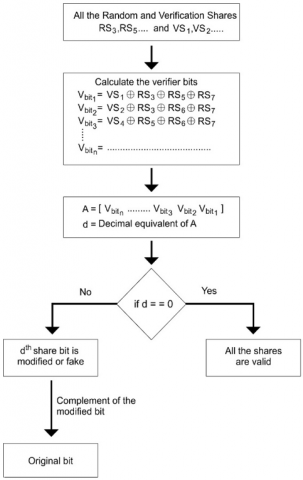Figure 2. Block diagram of SVC

Algorithm 1: Verification Share Generation (VSG)

Input: n random share of same size

Output: M verification share

Step 1: n=1;

For i= 1 to 2M-1

if (i ˄ (i-1)= = 0)

VSi=RS;

i++;

end

else

Si=SHn;

n++;

end

end

Step 2:

for k=0 to (m-1)

j=2k;

for i= 1 to 2M-1

if (Aj(i)= = 1)

VSj = VSj ⊕ Si;

end

i++;

end

k++;

end

Algorithm 2: Share Verification and Correction (SVC)

Input: M verification share and n random share

Output: Status of the shares as valid or fake and corrected modified/fake bit into original bit

Step 1:

For k=0 to (M-1)

n=M-1, j=2k;

for i= 1 to 2M-1

if (Aj(i)= = 1)

VSj = VSj ⊕ Si;

i++;

end

end

EM(n)=VSj;

n- -;

end

Step 2: Binary number which is stored in to EM, has been converted in to decimal number and if this decimal value (D) is equivalent to zero then all bits of all the shares are valid otherwise Dth share’s bit is modified/ fake.

Step 3: If in step 2, Dth share’s bit is found as modified/fake then the complimented value of modified/fake bit of Dth share will be an original bit.

## 3.png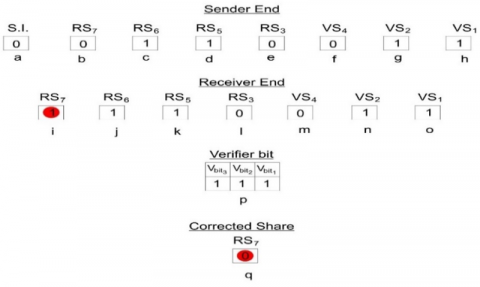Figure 3. Example of proposed VSG and SVC algorithms

Example 1: In this example, we have taken a secret image (black & white type image) of size 1 X 1. Suppose a (4, 4) visual secret sharing approach is applied, which generates the four random share (RSi). In this situation, three verification shares will be required to verify these random shares at the time of revealing the secret image. So, use the (7, 4) hamming code technique where the total number of shares is seven, and the random share of visual secret sharing is four, and three verification shares are used. Share numbers 3, 5, 6, and 7 are random shares (RSi), and remaining shares are verification shares (VSi), i.e., share numbers 1, 2, and 4. The verification shares are calculated as: VS1=RS3⊕RS5⊕RS7, VS2=RS3⊕RS6⊕RS7 and VS4=RS5⊕RS6⊕RS7 on the sender's side. All these shares are sent to legitimate users. The share verification process is done at the receiver end as follows-

Calculate the verifier bit

Vbit1= VS1⊕RS3⊕RS5⊕RS7

Vbit2= VS2⊕RS3⊕RS6⊕RS7

Vbit3= VS4⊕RS5⊕RS6⊕RS7

these all bits are stored in the array A= [Vbit3 Vbit2 Vbit1]

If all the bits of array A are zero, then all the shares are valid otherwise modified/fake share corresponding to the decimal value equivalent to the binary value stored in the array A. The above-discussed example is shown in Figure 3. In this example 7th, a random share is a fake/modified share. In this example, figure (a) shows the secret image (S.I.), (b)-(e) show the random share, (f)-(h) show verification share at sender end. Figure (i) –(l) show random share, (m) –(n) show verification share at the receiver end. Here, the verifier bit shows the 7th random share is fake share, and the corrected value of a fake share is shown in the Figure (q).

4. Experimental Results and Performance Analysis

4.1 Experimental results

4.1.1 Simulation 1

In the first simulation, we have taken a secret image (binary image type) of size 4 X 4. Suppose a (4, 4) visual secret sharing approach is applied, which generates the four random share (RSi). In this situation, three verification shares will be required to verify these random shares at the time of revealing the secret image. In Figure 4, (a) shows the secret image, (b)-(e) shows the random shares, (f)-(h) show the verification shares at the sender side and (i)-(l) show the random shares, (m)-(o) show the verification shares at the receiver end.

The verifier bits are calculated as follows

Vbit1= VS1⊕RS3⊕RS5⊕RS7

Vbit2= VS2⊕RS3⊕RS6⊕RS7

Vbit3= VS4⊕RS5⊕RS6⊕RS7

These all bits are shown in the figures (p), (q), (r), and (s) corresponding to the first, second, third, and fourth pixels of the shares. Figure (q) and (r) show the bit is genuine while (p) and (s) show that there are errors in a bit. The decimal value equivalent to the verifier bit present in the figure (p) and (s) is six and three, respectively. In this simulation, modified/ fake bits are found at the first and fourth pixels in the 6th and 3rd random share, respectively. The corrected pixels values (taken complement of error value) are shown in the figure (t) and (u) corresponding to 6th and 3rd random shares, respectively.

4.1.2 Simulation 2

In this simulation, the secret image of size 1 X 1 of black & white type has been taken for the (5, 11) VSS scheme, which generates the 11 random shares. Four number of verification shares are required to verify these random shares. Figure 4 shows the results of simulation 2. In Figure 5, (a) shows the secret image, (b)-(l) shows the random shares, (m)-(p) show the verification shares at the sender side and (b1)-(l1) show the random shares, (m1)-(p1)  show the verification shares at the receiver end. The verifier bits are shown in figure (q). The decimal value (D) of the verifier bit is not equivalent to the zero, it means that the error is present in the Dth random share. The third random share bit is a fake/modified bit, which is found in this simulation. The corrected bit is shown in figure (r). It is clear from the above discussion that the proposed scheme works effectively, and the accuracy of verifying and correcting ability of the fake share is 100%.

4.1.3 Simulation 3

In this simulation, a black & white type secret image of size 100 X 100 has been taken for the (4, 4) VSS scheme, which generates the four random shares (S1, S2, S3, S4). According to (4, 4) VSS scheme, all four shares are required for the reconstruction of the secret image; if the number of shares is less than four, then the reconstruction of the secret image is not possible. In this simulation, (4, 7) hamming code is applied for the share verification. Simulation results are shown in Figure 6. Figure 6, (a) shows the original secret image, (b)-(e) show the random shares, (f) shows the X-ORed result between S3 and S4, (g) shows the X-ORed result among S1, S2, and S3, (h) shows the X-ORed result among S2, S3, and S4, (i) shows the X-ORed result between S2 and S3, (j) shows the fake random share1 (FS1), (k) shows the X-ORed result among S1, S2, S3, and S4, (l) shows the X-ORed result among FS1, S2, S3, and S4. Figures k & l clearly show the difference between reconstructed secret images by using only genuine and genuine & fake shares, respectively. In this simulation, shares S2, S3, and S4 are found as genuine shares, while S1 is a fake/ modified share. The number of pixels modified in the share 1 (S1) is 121, which are shown in Table 1.

## 4.pngFigure 4. Results of simulation 1

## 5.png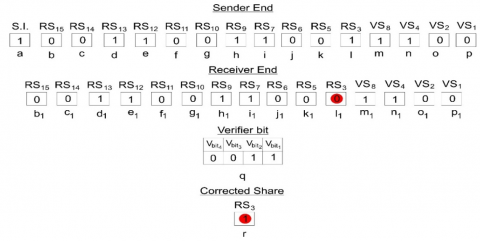Figure 5. Results of simulation 2

Table 1. Fake/modified number of pixels in simulation 3

 Shares Total no. of pixels Original no. of pixels Fake/Modified no. of pixels Share1 (S1) 10000 9879 121 Share2 (S2) 10000 10000 0 Share3 (S3) 10000 10000 0 Share4 (S4) 10000 10000 0
Table 2. Fake/modified number of pixels in simulation 4
 Shares Total no. of pixels Original no. of pixels Fake/Modified no. of pixels Share1 (S1) 65536 65195 341 Share2 (S2) 65536 65536 0 Share3 (S3) 65536 65230 306 Share4 (S4) 65536 65536 0

## 6.png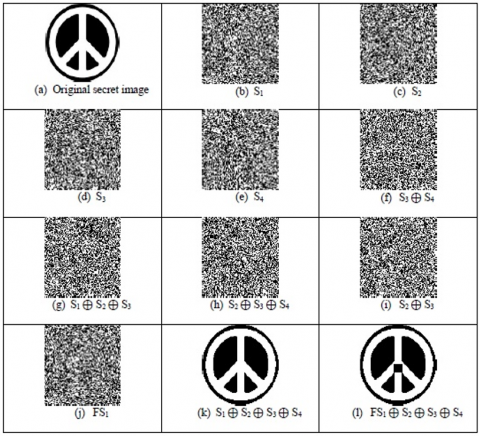Figure 6. Results of simulation 3

## 7.png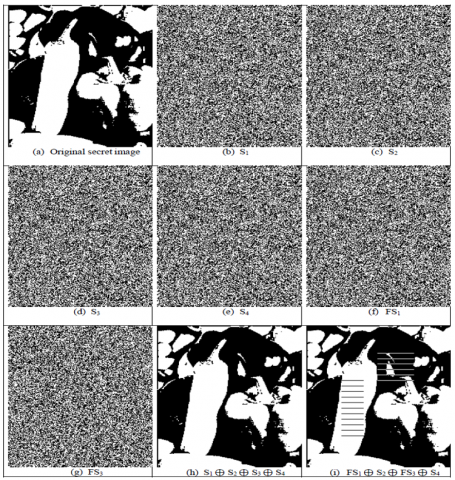Figure 7. Results of simulation 4

Table 3. Comparison between proposed scheme and related state of the arts

 Schemes Properties Number of secret shares Number of additional verification shares Size of shares Pixel expansion Contrast Conversion from modified share to original share Hu and Tzeng  n n Ws x Hs x (m+2) m+2 $\frac{|n w-n b|}{m+2}$ No Horng et al.  n n Ws x Hs x m - $\frac{|n w-n b|}{m}$ No Chen et al.  n n Ws x Hs x m - $\frac{|n w-n b|}{m}$ No Chen et al.  n n Ws x Hs x (m+t+1) m+t+1 $\frac{|n w-n b|}{m+t+1}$ No Lin et al.  n - Ws x Hs x m - $\frac{|1 / 2 n w-n b|}{m}$ No Proposed scheme n=2M -M-1 M Ws x Hs x m - $\frac{|n w-n b|}{m}$ Yes
4.1.4 Simulation 4

In this simulation, secret image of size 256 X 256 of black & white type have been taken for the (4, 4) VSS scheme which generates the 4 random shares (S1, S2, S3, S4). In (4, 4) VSS scheme, all four shares are required for the reconstruction of the secret image, if the number of shares are less than four, then the reconstruction of the secret image is not possible. In simulation 4, (4, 7) hamming code is applied for the share verification. Simulation results are shown in the Figure 7. Figure 7, (a) shows the original secret image, (b)-(e) show the random shares, (f)- (g) show the fake random share1 (FS1) and share3 (FS3), (h) shows the X-ORed result among S1, S2, S3 and S4, (i) shows the X-ORed result among FS1, S2, FS3 and S4. Figures h & i clearly show the difference between reconstructed secret images by using only genuine and genuine & fake shares respectively. In this simulation, shares S2 & S4 are found as the genuine shares while S1 & S3 are fake/ modified shares. The number of pixels modified in the shares S1 & S3 are 341 and 306 respectively which are shown in the Table 2.

4.2 Performance analysis

Proposed work has been compared with existed related researches on the basis of some important parameters which are shown in the Table 3. In the proposed scheme, M number of additional verification shares are required to verify n (n=2M-M-1) number of shares while in the other existed approaches, only M number of shares are verified. In example 1, a Hamming (7, 4) code was considered for explaining the proposed algorithms. However, this code is considered to be inefﬁcient because 4 random shares are verified by using 3 verification shares which are very close to the number of the random shares. In communication systems, they deﬁne a parameter called the code rate to quantify the efﬁciency of the code. The code rate is deﬁned as N/K. As the code rate approaches 1, this indicates that the code is more efﬁcient. In the case of (7, 4) code, the rate is N/K =4 /7=0 .571. However, it has been shown that using a number of verification shares M can serve a number of random shares N =2M −M −1, which renders the code rate N/K = 2M−M−1 / 2M−1. It can be seen that by increasing the number of verification shares M, the code rate approaches 1. For example, using M =6 can serve a number of random shares N = 57 giving a code rate of N/K = 57 /63 = 0.905. Increment in M (number of verification share) increases the code rate. Consequently, code becomes more efﬁcient than previous one. Further, pixel expansion is not required in the proposed work. On the basis of these two parameters, the proposed approach will require less computation and communication cost than other existed works [5-8]. Moreover, the proposed work has the ability to correct the modified bits in to the original bits while all the other existed cheating prevention approaches are not able to do so. The proposed scheme also does not affect the contrast of the reconstructed secret image. Contrast (α) is defined as the ratio of relative luminance difference of region on the superimposed shares which is created by a white pixel and a black pixel. It is used to measure the visual quality of the reconstructed secret image. High contrast is considered for the better approach. Contrast is formulated as follows-

$\alpha=\frac{|\mathrm{nw}-\mathrm{nb}|}{\mathrm{m}}$          (1)

where, nw and nb are the number of pixels in the region of white pixel and the region of a black pixel respectively and m is the pixel expansion.

## 8.png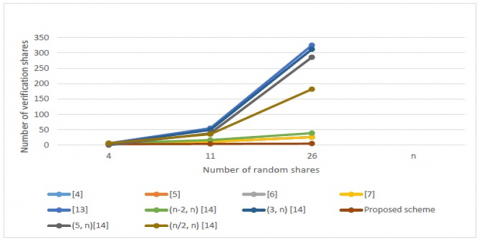Figure 8. Number of verification shares required for the (k, n) visual secret sharing scheme

From Table 3, it is clear that the proposed scheme is better than other approaches [5-9].

Table 4. Comparison between the proposed scheme and existed cheating prevention schemes

 Schemes Number of random shares Number of additional verification shares Recovery from modified share to the original share Lin et al.  n n*(n-1)/2 No Yang et al.  n n*(n-k+1)/2 No Proposed scheme n=2M -M-1 M Yes

Table 4 also shows the benefits of the proposed scheme compared to other approaches [14, 15]. The performance of the proposed scheme based on the required number of verification shares is shown in Figure 8. In this figure, it is clear that the proposed approach is efficient than the other existed methods [5-9, 14, 15].

5. Conclusions

In this paper, we have proposed a novel technique for cheating prevention and detection in visual secret sharing. This technique identifies the modified/fake bit of the shares. Moreover, it retains the originality of bit by correcting the modified bit. The proposed work can be applied on any visual secret sharing for cheating prevention. The proposed scheme is more efficient in terms of pixel expansion and requires less number of additional verification shares than the other existing cheating prevention schemes. It also does not affect the contrast of the reconstructed secret image. The theoretical and experimental analysis of the work shows its effectiveness.  The accuracy of retaining the genuine bits from identified fake/modified bits is 100%.

References

 Yan, W.Q., Jin, D., Kankanhalli, M.S. (2004). Visual cryptography for print and scan applications. In Proceedings of International Symposium on Circuits and Systems, Vancouver, BC, Canada, pp. 572-575. https://doi.org/10.1109/ISCAS.2004.1329727

 Subba Rao, Y.V., Sukonkina, Y., Bhagwati, C., Singh, U.K. (2008). Fingerprint based authentication application using visual cryptography methods. TENCON 2008 - 2008 IEEE Region 10 Conference, Hyderabad, India. https://doi.org/10.1109/TENCON.2008.4766425

 Naor, M., Shamir, A. (1994). Visual cryptography. In: De Santis A. (eds) Advances in Cryptology — EUROCRYPT'94. EUROCRYPT 1994. Lecture Notes in Computer Science, vol 950. Springer, Berlin, Heidelberg. https://doi.org/10.1007/BFb0053419

 Kafri, O., Keren, E. (1987). Encryption of pictures and shapes by random grids. Optics Letters, 12(6): 377-379. https://doi.org/10.1364/OL.12.000377

 Horng, G.B., Chen, T.H., Tsai, D.S. (2007). Cheating in visual cryptography. Designs, Codes and Cryptography, 38: 219-236. https://doi.org/10.1007/s10623-005-6342-0

 Hu, C.M., Tzeng, W.G. (2006). Cheating prevention in visual cryptography. IEEE Transactions on Image Processing, 16(1): 36-45. https://doi.org/10.1109/TIP.2006.884916

 Chen, Y.C., Tsai, D.S., Horng, G.B. (2012). A new authentication based cheating prevention scheme in Naor-Shamirs visual cryptography. Journal of Visual Communication and Image Representation, 23(8): 1225-1233. https://doi.org/10.1016/j.jvcir.2012.08.006

 Chen, Y.C., Horng, G.B., Tsai, D.S. (2012). Comment on cheating prevention in visual cryptography. IEEE Transactions on Image Processing, 21(7): 3319-3322. https://doi.org/10.1109/TIP.2012.2190082

 Lin, P.Y., Wang, R.Z., Chang, Y.J., Fang, W.P. (2015). Prevention of cheating in visual cryptography by using coherent patterns. Information Sciences, 301: 61-74. http://doi.org/10.1016/j.ins.2014.12.046

 Yang, C.N., Cimato, S., Wu, J.H., Cai, S.R. (2016). 3-out-of-n cheating prevention visual cryptographic schemes. ICISSP 2016 (International Conference on Information Systems Security and Privacy), Proc. of ICISSP, Rome, Italy, pp. 400-406. http://doi.org/10.5220/0005740504000406

 Ranvijay, M.Y. (2019). Verifiable essential secret image sharing with multiple decryption. International Journal of Recent Technology and Engineering, 8(2): 2211-2220. http://doi.org/10.35940/ijrte.B2424.078219

 Ranvijay, M.Y. (2019). Collusion attacks in XOR based RG-VSS. International Journal of Innovative Technology and Exploring Engineering, 8(10): 2339-2345. http://doi.org/10.35940/ijitee.J8783.0881019

 Hamming, R.W. (1950). Error detecting and error correcting codes. The Bell System Technical Journal, 29(2): 147-160. https://doi.org/10.1002/j.1538-7305.1950.tb00463.x

 Lin, C.H., Chen, T.H., Wu, Y.T., Tsao, K.H., Lin, K.S. (2014). Multi-factor cheating prevention in visual secret sharing by hybrid codebooks. Journal of Visual Communication and Image Representation, 25(7): 1543-1557. http://doi.org/10.1016/j.jvcir.2014.06.011

 Yang, C.N., Wu, F.H., Peng, S.L. (2018). Enhancing multi-factor cheating prevention in visual cryptography based minimum (k, n)-connected graph. Journal of Visual Communication and Image Representation, 55: 660-676. http://doi.org/10.1016/j.jvcir.2018.07.012